Anzeige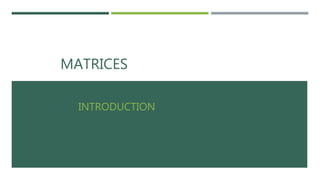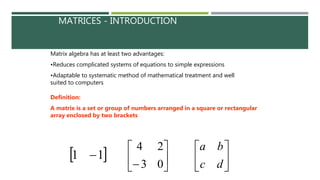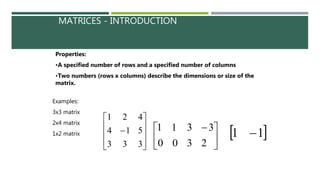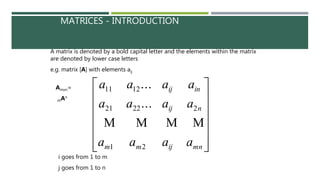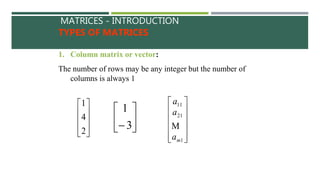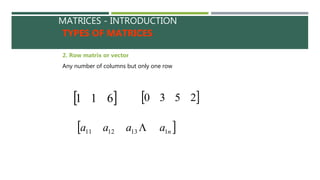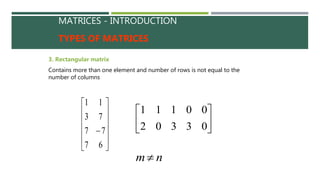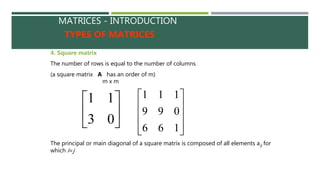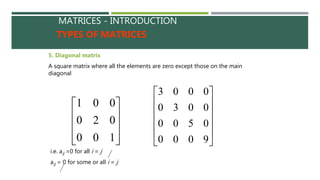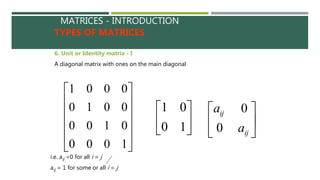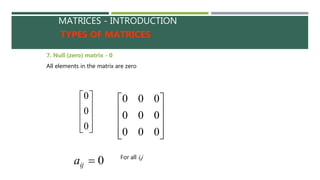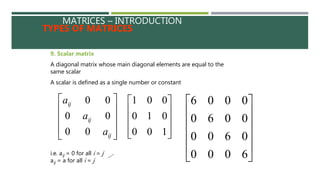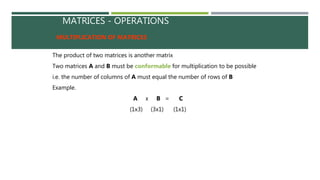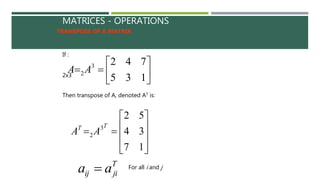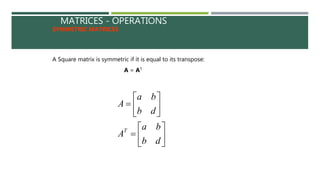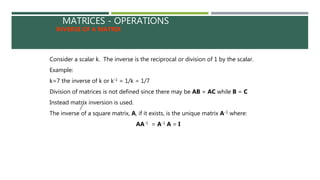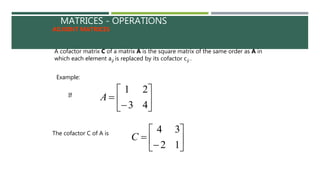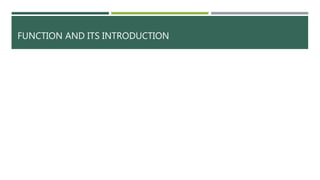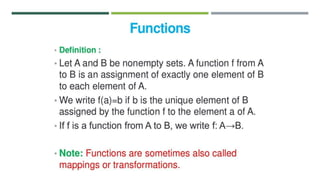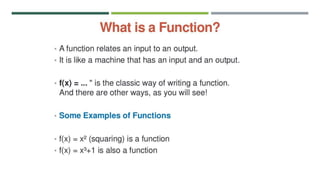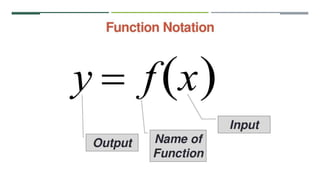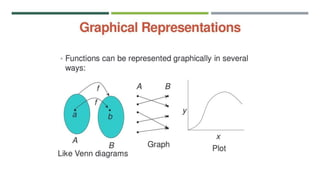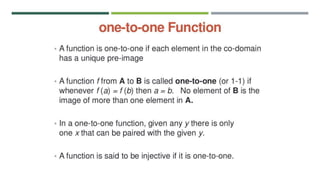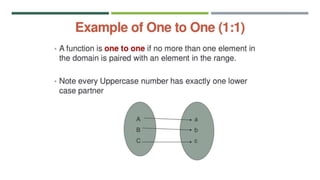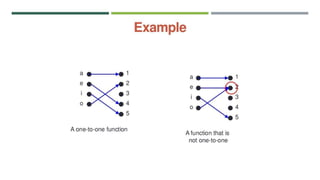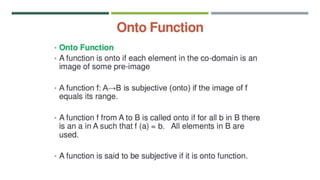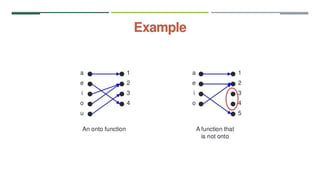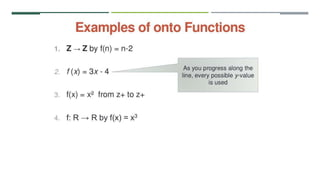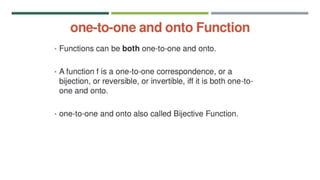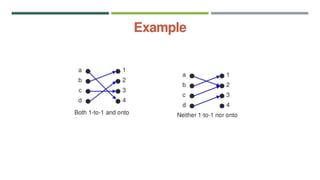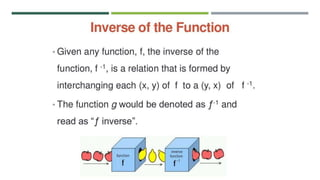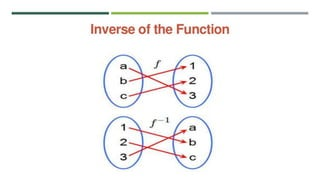1 von 33
Anzeige

### matrices and function ( matrix)

1. WELCOME TO MY PRESENTATION  SUBMITTED BY: REZA AT-TANZIL ID:11915037 DEPT. OF PHARMACY SESSION:2018-19 COMILLA UNIVERSITY SUBMITTED TO: JOY CHARNDRA RAJBONGSHI LECTURER,DEPT. OF PHARMACY COMILLA UNIVERSITY
2. MATRICES INTRODUCTION
3. MATRICES - INTRODUCTION Matrix algebra has at least two advantages: •Reduces complicated systems of equations to simple expressions •Adaptable to systematic method of mathematical treatment and well suited to computers Definition: A matrix is a set or group of numbers arranged in a square or rectangular array enclosed by two brackets  11         03 24       dc ba
4. MATRICES - INTRODUCTION Properties: •A specified number of rows and a specified number of columns •Two numbers (rows x columns) describe the dimensions or size of the matrix. Examples: 3x3 matrix 2x4 matrix 1x2 matrix            333 514 421        2 3 3 3 0 1 0 1  11 
5. MATRICES - INTRODUCTION A matrix is denoted by a bold capital letter and the elements within the matrix are denoted by lower case letters e.g. matrix [A] with elements aij               mnijmm nij inij aaaa aaaa aaaa 21 22221 1211 ... ...  i goes from 1 to m j goes from 1 to n Amxn= mAn
6. MATRICES - INTRODUCTION TYPES OF MATRICES 1. Column matrix or vector: The number of rows may be any integer but the number of columns is always 1           2 4 1       3 1             1 21 11 ma a a 
7. MATRICES - INTRODUCTION TYPES OF MATRICES 2. Row matrix or vector Any number of columns but only one row  611  2530  naaaa 1131211 
8. MATRICES - INTRODUCTION TYPES OF MATRICES 3. Rectangular matrix Contains more than one element and number of rows is not equal to the number of columns              67 77 73 11       03302 00111 nm
9. MATRICES - INTRODUCTION TYPES OF MATRICES 4. Square matrix The number of rows is equal to the number of columns (a square matrix A has an order of m)       03 11           166 099 111 m x m The principal or main diagonal of a square matrix is composed of all elements aij for which i=j
10. MATRICES - INTRODUCTION TYPES OF MATRICES 5. Diagonal matrix A square matrix where all the elements are zero except those on the main diagonal           100 020 001             9000 0500 0030 0003 i.e. aij =0 for all i = j aij = 0 for some or all i = j
11. MATRICES - INTRODUCTION TYPES OF MATRICES 6. Unit or Identity matrix - I A diagonal matrix with ones on the main diagonal             1000 0100 0010 0001       10 01 i.e. aij =0 for all i = j aij = 1 for some or all i = j       ij ij a a 0 0
12. MATRICES - INTRODUCTION TYPES OF MATRICES 7. Null (zero) matrix - 0 All elements in the matrix are zero           0 0 0           000 000 000 0ija For all i,j
13. MATRICES – INTRODUCTION TYPES OF MATRICES 9. Scalar matrix A diagonal matrix whose main diagonal elements are equal to the same scalar A scalar is defined as a single number or constant           100 010 001             6000 0600 0060 0006 i.e. aij = 0 for all i = j aij = a for all i = j           ij ij ij a a a 00 00 00
14. MATRICES - OPERATIONS MULTIPLICATION OF MATRICES The product of two matrices is another matrix Two matrices A and B must be conformable for multiplication to be possible i.e. the number of columns of A must equal the number of rows of B Example. A x B = C (1x3) (3x1) (1x1)
15. MATRICES - OPERATIONS TRANSPOSE OF A MATRIX If :        135 7423 2AA2x3            17 34 52 3 2 TT AA Then transpose of A, denoted AT is: T jiij aa  For all i and j
16. MATRICES - OPERATIONS SYMMETRIC MATRICES A Square matrix is symmetric if it is equal to its transpose: A = AT               db ba A db ba A T
17. MATRICES - OPERATIONS INVERSE OF A MATRIX Consider a scalar k. The inverse is the reciprocal or division of 1 by the scalar. Example: k=7 the inverse of k or k-1 = 1/k = 1/7 Division of matrices is not defined since there may be AB = AC while B = C Instead matrix inversion is used. The inverse of a square matrix, A, if it exists, is the unique matrix A-1 where: AA-1 = A-1 A = I
18. MATRICES - OPERATIONS ADJOINT MATRICES A cofactor matrix C of a matrix A is the square matrix of the same order as A in which each element aij is replaced by its cofactor cij . Example:         43 21 A         12 34 C If The cofactor C of A is
19. FUNCTION AND ITS INTRODUCTION
Anzeige